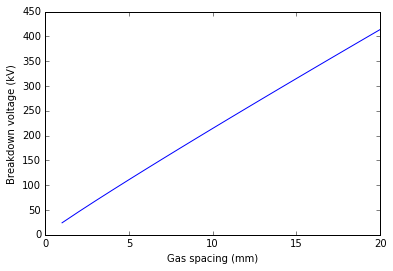# Chapter 3: Conduction and Breakdown in Liquid Dielectrics¶

## Example 1: pg 85¶

In :
#example 3.1
#determination of power law dependence between the gap spacing  and the applied voltage of the oil
%matplotlib inline
from math import log, exp
from matplotlib import pyplot
import numpy as np
#given data
d1=4.#gap spacing(in mm)
d2=6.#gap spacing(in mm)
d3=10.#gap spacing(in mm)
d4=12.#gap spacing(in mm)
V1=90.#voltage(in kV) at breakdown
V2=140.#voltage(in kV) at breakdown
V3=210.#voltage(in kV) at breakdown
V4=255.#voltage(in kV) at breakdown

#calculation
#from the relationship between breakdown voltage and the gap spacing.....V = K*d^n
#we get n = (log(V)-log(K))/log(d) = slope of line from given data
n=(log(V4)-log(V1))/(log(d4)-log(d1))
K=exp(log(V1)-n*log(d1))#Y intercept on the power law dependence graph
#plotting of graph
dn=np.linspace(1,20,num=20)
Vn=K*dn**n
#results
pyplot.plot(dn,Vn)
pyplot.xlabel("Gas spacing (mm)")
pyplot.ylabel("Breakdown voltage (kV)")
pyplot.show()
print 'The power law dependence between the gap spacing  and the applied voltage of the oil is ',round(K,1),'*d^',round(n,3)The power law dependence between the gap spacing  and the applied voltage of the oil is  24.2 *d^ 0.948